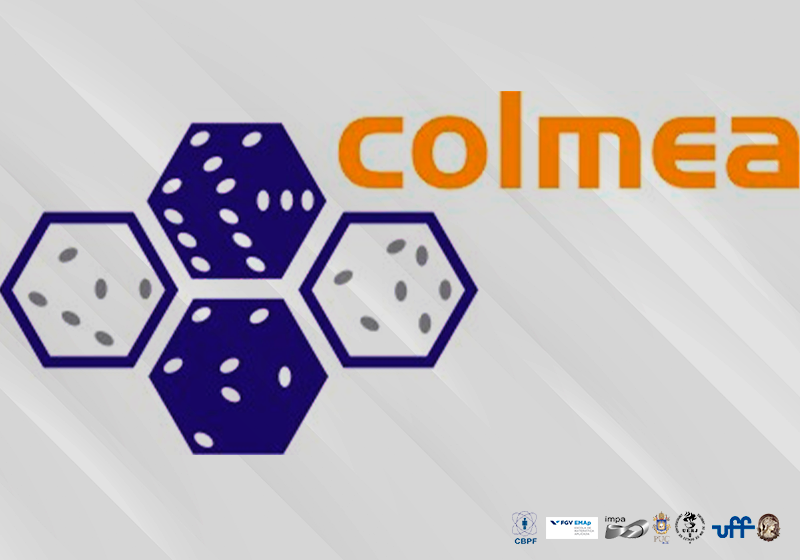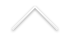Data: 22/11/2023
Horário: a partir das 14:00h
Local: Sala de reuniões do Decanato do CTC, 12 º andar do prédio Cardeal Leme, PUC-Rio

Nesta ocasião teremos palestras de Philip Thompson (FGV EMAp)  e Oliver Riordan (Oxford).

Programa:

14:00 h - 15:20h - Philip Thompson (FGV EMAp)
Outlier-robust additive matrix decomposition and robust matrix completion

Abstract: We study least-squares trace regression when the parameter is the sum of a $r$-low-rank matrix with a $s$-sparse matrix and a fraction $\epsilon$ of the labels is corrupted. For subgaussian distributions, we highlight three needed design properties, each one derived from a different process inequality: the product process inequality'', Chevet's inequality'' and the multiplier process inequality''. Jointly, these properties entail the near-optimality of a tractable estimator with respect to the effective dimensions for the low-rank and sparse components, $\epsilon$ and the failure probability $\delta$. ... Our estimator is adaptive to $(s,r,\epsilon,\delta)$ and, for fixed absolute constant $c>0$, it attains the mentioned rate with probability $1-\delta$ uniformly over all $\delta > exp(-cn)$. Disconsidering matrix decomposition, our analysis also entails optimal bounds for a robust estimator adapted to the noise variance. Finally, we consider robust matrix completion. We highlight a new property for this problem: one can robustly and optimally estimate the incomplete matrix regardless of the magnitude of the corruption. Our estimators are based on sorted'' versions of Huber's loss. We present simulations matching the theory. In particular, it reveals the superiority of sorted'' Huber's losses over the classical Huber's loss.

15:40h - 17:00h - Oliver Riordan (Oxford)
The chromatic number of random graphs

17:00h - Discussão e lanche

Todos são muito bem-vindos.

Informações mais completas sobre o COLMEA podem ser encontradas AQUI.